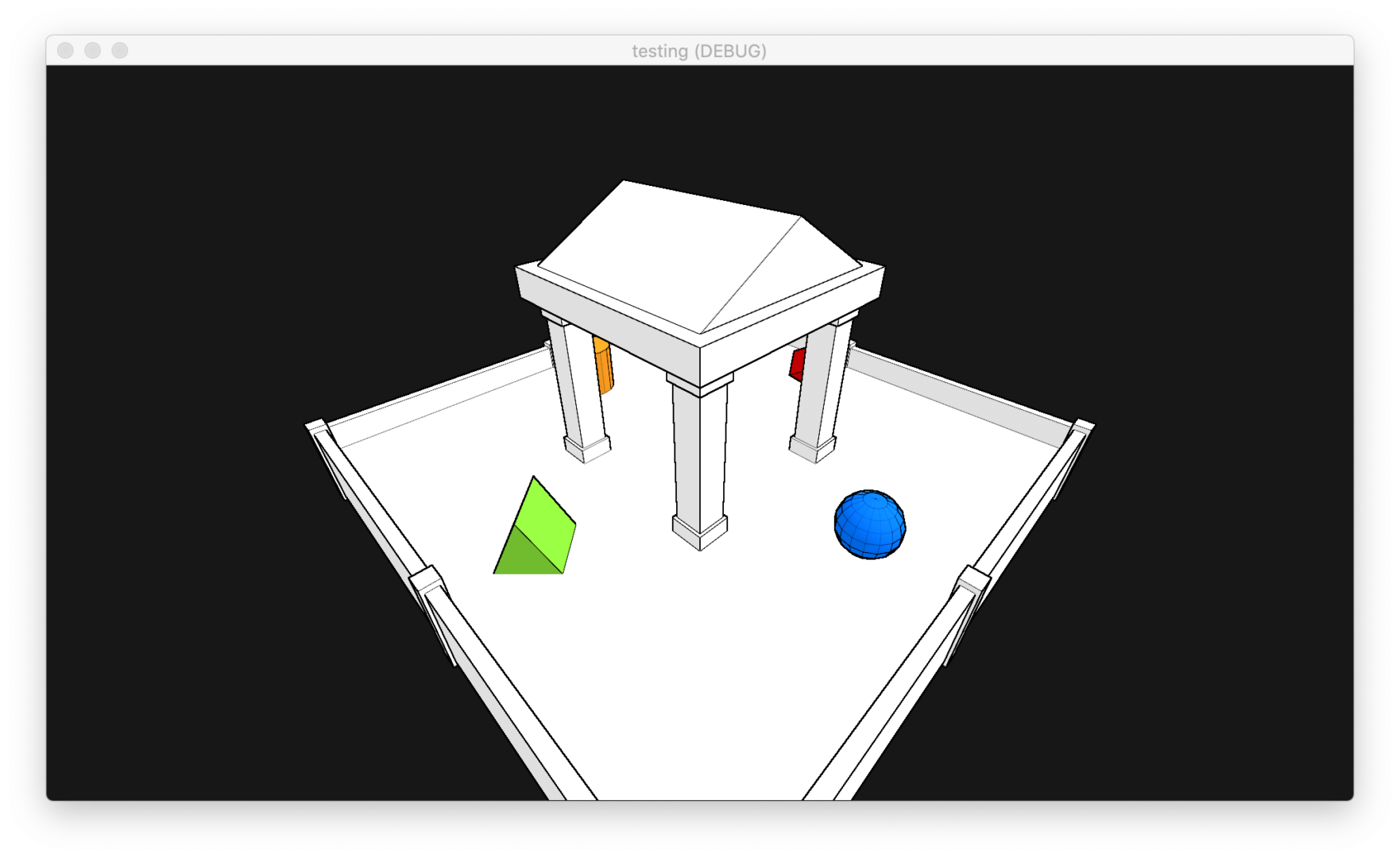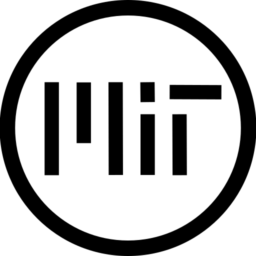# Screen-Space Edge Detection Outline Shader

This shader must be applied to a Quad (MeshInstance) with a size of (2, 2)!

Extra Cull Margin on the quad should be turned up all the way!

This shader gets the depth information for the current pixel and the pixels around it, then instead of just comparing the difference between the depths (this would only give you outlines on the outside of objects, it’s how I did outline_mode 1) It first finds the difference between the center pixel and those around it, then compares the differences to the differences from the opposite side (this easily detects drastic changes in the angle of the depth, ie. edges)

You can change the color of the outline in the Shader Params.

You actually can’t change the width of the outline in this shader though, widening the outline would require lots more computation.

If you’d like to support my work, consider donating some crypto!

bitcoin: bc1qdsmmjp3az6dvr5u00ffxflzuth87zsv7ld4xcg

ethereum: 0x5748889bE74F3012543d82160Fa3E6fBe55a3bff

dogecoin: DSbSHKkwsLE35Bos2vpwxwthXGa9EoP8rB

``````//THIS SHADER MUST BE APPLIED TO A QUAD (MeshInstance) WITH A SIZE OF (2, 2)
//Extra Cull Margin on the quad should be turned up all the way!

uniform int outline_mode : hint_range(1, 3, 1) = 3;
uniform float outline_intensity : hint_range(0, 5) = 1;
uniform bool _round = false;
uniform float outline_bias : hint_range(-10, 10) = 0;

uniform vec4 outline_color : hint_color = vec4(0.0, 0.0, 0.0, 1.0);

void vertex() {
POSITION = vec4(VERTEX, 1.0);
}

void fragment() {
ALBEDO = outline_color.rgb;

vec2 screen_size = vec2(textureSize(SCREEN_TEXTURE, 1));

float px = 0.5/screen_size.x;
float py = 0.5/screen_size.y;

float d = texture(DEPTH_TEXTURE, SCREEN_UV).x;
float du = texture(DEPTH_TEXTURE, SCREEN_UV+vec2(0.0, py)).x;
float dd = texture(DEPTH_TEXTURE, SCREEN_UV+vec2(0.0, -py)).x;
float dr = texture(DEPTH_TEXTURE, SCREEN_UV+vec2(px, 0.0)).x;
float dl = texture(DEPTH_TEXTURE, SCREEN_UV+vec2(-px, 0.0)).x;

if (outline_mode == 1){
ALPHA = 0.0 + abs(abs(d)-abs(du)) + abs(abs(d)-abs(dd)) + abs(abs(d)-abs(dl)) + abs(abs(d)-abs(dr));

ALPHA *= 1000.0*outline_intensity;
} else if (outline_mode == 2) {
ALPHA = 0.0 + abs(abs(abs(d)-abs(du)) - abs(abs(d)-abs(dd))) + abs(abs(abs(d)-abs(dl)) - abs(abs(d)-abs(dr)));

ALPHA *= 3.0*50000.0*outline_intensity;
} else if (outline_mode == 3) {
float dq = texture(DEPTH_TEXTURE, SCREEN_UV+vec2(-px, py)).x;
float de = texture(DEPTH_TEXTURE, SCREEN_UV+vec2(px, py)).x;
float dz = texture(DEPTH_TEXTURE, SCREEN_UV+vec2(-px, -py)).x;
float dc = texture(DEPTH_TEXTURE, SCREEN_UV+vec2(px, -py)).x;

ALPHA = 0.0 + abs(abs(abs(d)-abs(du)) - abs(abs(d)-abs(dd))) + abs(abs(abs(d)-abs(dl)) - abs(abs(d)-abs(dr))) + abs(abs(abs(d)-abs(dq)) - abs(abs(d)-abs(dc))) + abs(abs(abs(d)-abs(dz)) - abs(abs(d)-abs(de)));

ALPHA *= 50000.0*outline_intensity;
}

ALPHA += outline_bias;

if (_round) {
ALPHA = round(ALPHA);
}

ALPHA *= outline_color.a;
}

//Written by Warren Jennings``````
###### Tags
outline, screen-spaceThe shader code and all code snippets in this post are under MIT license and can be used freely. Images and videos, and assets depicted in those, do not fall under this license. For more info, see our License terms.

### Cheaper* Edge Detection Post-ProcessingInline Feedbacksvoxibit
1 year ago

Awesome shader, thanks for sharing! One thing I added at the end was

```ALPHA = clamp(ALPHA, 0.0, 1.0);
```

For my scene I set the bias very negative, which was causing the picture to become overexposed until I clamped alpha.ColorauGuiyino
10 months ago

This shader is simply AWESOME!
Thank you.Filipi Brian
2 months ago

Hi, good morning, very amazing shader, thanks for sharing the same, how can I do this directly for a single object, I’m creating the first shaders, and I would like to implement this contour method if it’s not a clear problem?